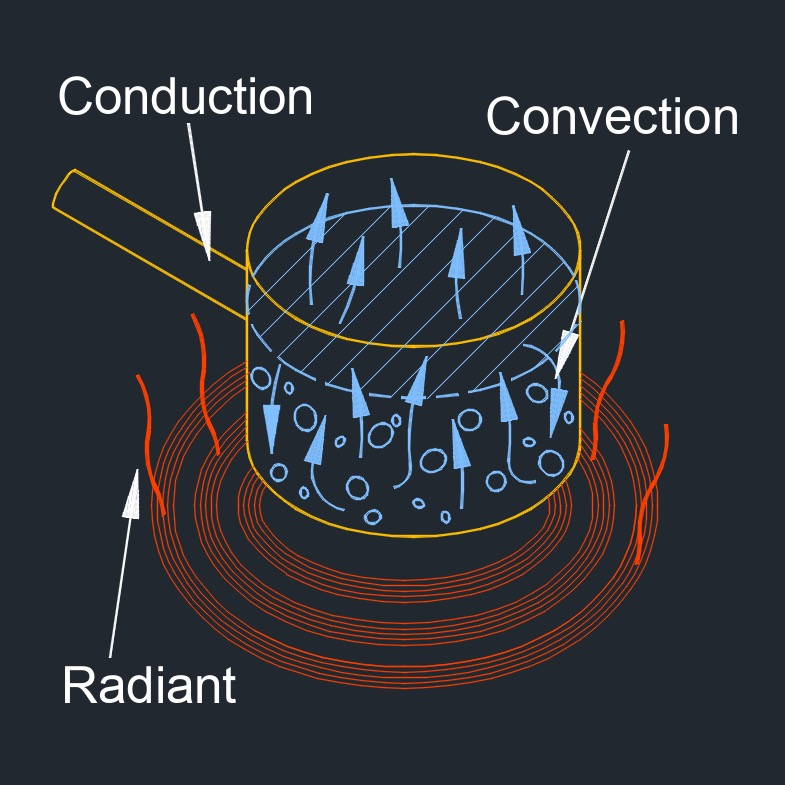# Heat Transfer by Conduction

on . Posted in ThermodynamicsHeat transfer by conduction, abbreviated as Qc, also called heat conduction or conduction heat transfer, is one of four types of heat transfer: conduction, convection, mixing and radiation. It is the second most efficient form of heat transfer after mixing. It is the flow of energy between two objects, or within one object, where there is a temperature differential.  Energy flow is from high energy to low. (Hot to cold)

Common thermal conductivity constants for non-metallic construction materials can be found here.

## Heat transfer by conduction formula

$$\large{ Q_c = \frac { k \; A_c \; \left( T_h \;-\; T_c \right) } {d} }$$
Symbol English Metric
$$\large{ Q_c }$$ = heat transfer by conduction $$\large{\frac{Btu}{hr}}$$  $$\large{ W }$$
$$\large{ A_c }$$ = area cross-section $$\large{ in^2 }$$ $$\large{ mm^2 }$$
$$\large{ T_c }$$ = cooler temperature $$\large{ F }$$ $$\large{ K }$$
$$\large{ T_h }$$ = higher temperature $$\large{ F }$$ $$\large{ K }$$
$$\large{ k }$$ = thermal conductivity $$\large{\frac{Btu-ft}{hr-ft^2-F}}$$  $$\large{\frac{W}{m-K}}$$
$$\large{ d }$$ = thickness of the material $$\large{ in }$$ $$\large{ mm }$$